## Math Teaching strategy #4: Teach only one procedure at a time

### It’s far better to know only one way to get there, than to get lost every time!

There are educational gurus out there promoting the idea that by giving students multiple solution paths it will give them a deeper understanding of math.  Generally these experts know this from teaching pre-service teachers in college, some of whom come to have some insights by learning multiple paths to the same goal.  Sorry folks.  What works for pre-service teachers in college, does not [and never will] apply to most children.

True, there are multiple ways to solve most arithmetic problems.  They have been discovered over centuries across multiple civilizations.  While one might dream of knowing all the ways to do long division, it’s far better to have one reliable method learned than to simply be confused and to get lost each time.  Just as in directions to go someplace, it is hard to remember all the steps in the directions.  When you’re new to a destination, the lefts and the rights are all arbitrary.  If you get two or more sets of directions, you are going to mix the steps from one way with the steps from the other method and you will not arrive at your destination.

## Math teaching strategy: Teach one solution method and stick to that until everyone has it mastered.

In real life, as in math, once you learn one reliable method of getting to your destination, you are then free to learn additional ways, or to try short cuts.  But please don’t confuse a beginning learner with short cuts or alternative methods.  It adds to the memory load and there are additional things to think about when trying alternatives.  Before the learner is solid in one method, the new information is likely going to get mixed up with the not-yet-learned material, leading to missteps and getting lost. Teach the long way every time and leave them to finding the short cuts on their own time.

But teachers say, “I want them to have a holistic understanding of what they are doing!”  Which is laudable, but that understanding has to come AFTER a reliable set of procedures is mastered.  There’s no reason that additional learning can’t be added to the student’s knowledge base, but it can’t come before or in place of learning a simple, basic, reliable procedure.   These admirable goals of getting a deeper understanding of math are fine, but they require MORE teaching than what used to be done, back when we were only taught algorithms for arithmetic.  There is time to learn more than the algorithms, if we teach effectively and efficiently.  Unfortunately, the deeper and more profound understandings in math can’t precede or be substituted for teaching the algorithms.

If you don’t believe me, ask a typical middle school student to do some arithmetic for you these days.  Few of them have mastered any reliable procedures for doing long division or converting mixed numbers or adding unlike fractions, etc.  It’s time to accept that teaching one way of doing things is better than none.

## Math Teaching strategies #5: Separate the introduction of similar concepts

### Teaching two similar concepts and their vocabulary terms at the same time creates confusion.

The classic example is teaching parallel and perpendicular on the same day.  The two concepts have to do with orientation of lines and the new vocabulary terms for them are similar.  So teaching them at the same time means some or many students will have the two terms confused for a long time.  That is known as a chronic confusion–possibly permanent.  They will know that orientation of lines is one of those two terms, possibly, but will be confused about which is which.  The predictable conversation with the teacher goes thusly:Teacher:  See these two lines lines.  Are they parallel or perpendicular?

Student: I think they’re perpendicular.

Teacher: Well…

Student: No, wait! I know.  They’re parallel.

Teacher: Yes, you’re right.  I’m glad you’ve learned that.

In case you missed it, the above student did NOT know the correct term.  The student just knew it was one of two terms, but unsure as to which one.  So the student picked one and as soon as there wasn’t confirmation by the teacher, switched to the other term.  When you’re busy teaching it is easy to get fooled by that kind of response into assuming the students really did learn it.

Other examples abound in math.  Teaching numerator and denominator in the same lesson is common.  Teaching the terms proper fractions and improper fractions on the same day is another example.  Acute and obtuse angles are yet another pair of chronically confused concepts that are introduced simultaneously.

## Math teaching strategies: Separate the introduction of similar concepts in time.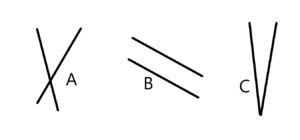If you teach one concept and only one of the pair, there’s no cause for confusion.  It will still take a lot of repetition and practice for it to be cemented into memory, but students will be clear.  If they use the term, for example parallel a lot of times in conjunction with examples they will soon (in a couple of weeks) be able to recall.  However, you should pair the concept with non-examples of the concept.  Not the opposite necessarily but just not an example of the concept.

For example: For the picture to the right you would ask the students.

A “Are the two lines in item A parallel or not parallel?”   Ans: Not parallel.

B “Are the two lines in item B parallel or not parallel?”   Ans: Parallel.

C “Are the two lines in item C parallel or not parallel?”   Ans: Not parallel.

Then after a couple of weeks you could introduce perpendicular.  Again teach it on its own and then contrasted with non-examples until the vocabulary was clear. Probably for a couple of weeks.   Only then can you combine both terms in the same lesson.

Another advantage of this approach is that you have avoided the other common mistake.  Students sometimes come to the conclusion that all pairs of lines are either parallel or perpendicular.  This method of introducing the concepts helps them realize that there are non-examples of each concept, parallel lines and ones that aren’t as well as perpendicular lines and ones that aren’t as well.

## Math teaching strategies #6: Teaching a new concept using a common sense name

Here’s an example of the problem.   A brachistochrone (pictured above) is a curve between two points along which a body can move under gravity in a shorter time than for any other curve. It is the same curve as a cycloid, but just hanging downward.   A cycloid is the path traced by a point on a wheel as the wheel rolls, without slipping, along a flat surface. The standard parametrization is x = a(t – sin t),y = a(1 – cos t), where a is the radius of the wheel.Introducing this concept and the term brachistochrone at the same time would be designed so the teacher can use brachistochrone and its concept in instruction.  However, because the term is new and the concept is also new, when the teacher uses the new term during later instruction, the students will have difficulty bringing the concept into their minds. “So a brachistochrone has some other cool properties. What’s the primary thing we know about the brachistochrone?”

Instruction not working.  If you watch instruction where the term and the concept have been taught simultaneously, confusion ensues when the teacher uses the term.  You’ll see students looking away as they try to bring up the explanation of that weird new term in their memory (see the pictured example?).  Sometimes the teacher will notice this and give a thumbnail definition or example of the term, and the students will then remember.  However, the teacher should then realize that the concept was not connected to the new vocabulary term.

## Math teaching strategies: Teach new concepts using a common sense term at first.

When a new vocabulary term is used to introduce a new concept, students will need a lot of practice with recalling the term and the definition before it can be used in instruction.  On the other hand, students can quickly understand and use new concepts and ideas in math if they don’t have to learn a new word for it.  Using a common sense term, the idea or concept can be worked with, the implications studied and it can be applied to real world problems almost immediately.  Students can later quite easily learn proper vocabulary terms for concepts they understand and recognize.  Here’s an example.

The “shortest time curve.”  It is more efficient and effective to teach the concept first and use a common sense term for it.  I would call a brachistochrone the “shortest time curve.”**  Instruction would proceed with the, “Do you remember that ‘shortest time curve’ we talked about last week?”  Students would easily be able to remember it.  Instruction would go like this: “So ‘the shortest time curve’ has some other cool properties. What’s the primary thing we know about the ‘shortest time curve’?”  Students would easily be able to answer this question.

Then after students have worked with the concept of “the shortest time curve” for a couple of weeks, you can add the vocabulary term to it. “By the way, the proper mathematical name for “the shortest time curve” is called a brachistochrone. Isn’t that cool?”  Students will want to learn its proper name as a point of pride about knowing this fancy term for a concept they already “own,” rather than a point of confusion.

**Actually that’s what brachistochrone means in Greek: brakhistos, meaning shortest and khronos meaning time.

## Learning to Add Integers

### Four Problem Types to Learn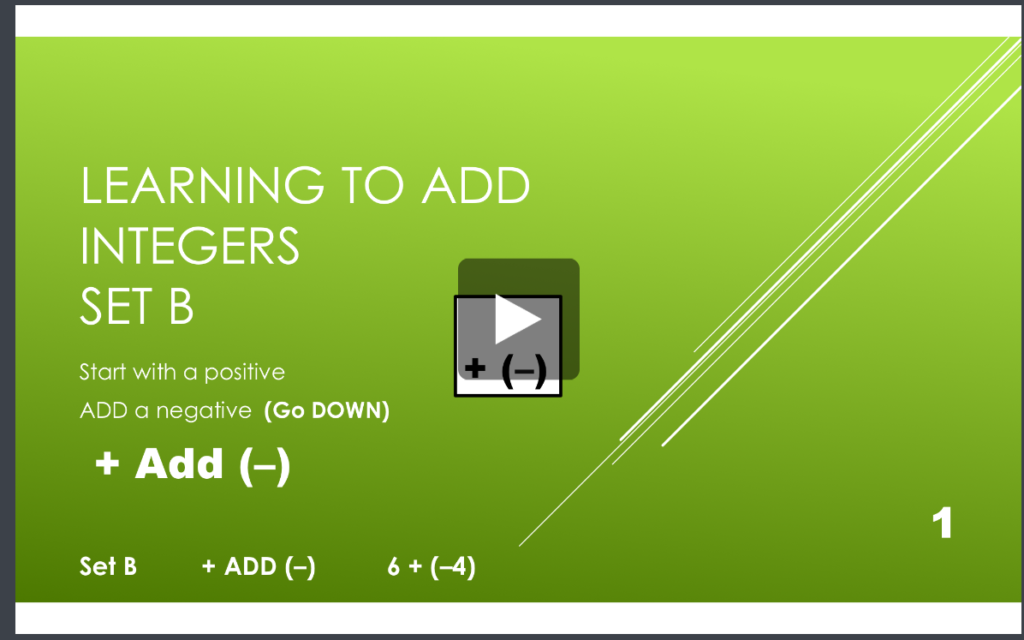Learning to Add Integers displays problems on a vertical number line and then teaches students two rules about how to solve problems that add positive and negative numbers.
Rule 1:  When you add a positive number, go UP.
Rule 2:  When you add a negative number, go DOWN.

Click to see online lesson.  Doing problems on the vertical number line is more intuitively appealing because UP is more and DOWN is always less.  This makes crossing zero a little easier to comprehend.

Students learn how these two rules play out with two types of problems: when starting with a positive number and when starting with a negative number. Students gradually learn all four types of problems.  On each worksheet they see how to solve each problem type using the number line working with their partner.  Then students learn to recognize the pattern of each problem type by orally answering several examples of each type with their partner (going around the outside of the page).  You will probably not be surprised that there is a one-minute test on each set.   Students are to be 100% accurate and to meet or beat their goal from the special writing speed test for Learning to Add integers (the fastest goal is only 28 problems in a minute).

Students can watch 4 online lessons which teach how each type of problem is solved and why it is correct.

(1) Add Integers Set A Positive add a positive

(2) Add Integers Set B Positive add a negative

(3) Add Integers Set G Negative add a negative

(4) Add Integers Set L Negative add a positive

## Are your students wary of working with integers?

Many students find integers confusing.  If you add a negative to a negative are you getting more or less??? Over the years different “rules” have been used to try to remember what should happen.  Rules such as “two negatives make a plus” or “opposite signs subtract.”  Whatever is used to try to remember, it interferes with a student’s ability to quickly and reliably get the answers without having to stop and puzzle it out.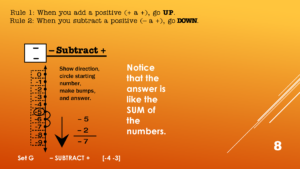I have posted a series of free lessons online (links below) that use a vertical number line to take some of the confusion out of the process.  Turns out there are a total of eight types of problems but all of them can be solved with the same process on the vertical number line.  Intuitively on a vertical number line, up is more and down is less.

(1) Mixed Integers Set A1 Positive add a positive

(2) Mixed Integers Set A2 Positive subtract a positive

(3) Mixed Integers Set D Negative add a positive

(4) Mixed Integers Set G Negative subtract a positive

(5) Mixed Integers Set J Negative subtract a negative

(6) Mixed Integers Set M Positive subtract a negative

(7) Mixed Integers Set P Positive add a negative

(8) Mixed Integers Set S Negative add a negative

Using the vertical number line there are two rules to learn.  Rule 1: When you add a positive or subtract a negative you go up on the number line.  Rule 2: When you subtract a positive or add a negative you go down on the number line.

So first thing to figure out is whether you’re going up or down.  Once you do that you simply make “bumps” going either up or down from where you start.  That gives you the answer without any uncertainty.  These lessons are quick (about 2 minutes) and identify a pattern of whether the answer is like the sum or the difference between the numbers.  Once students can recognize the pattern they can begin to answer fluently and without a struggle.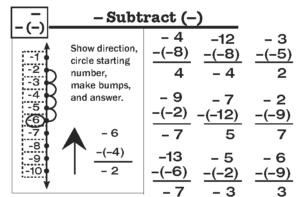To help with the work of learning to quickly and easily recognize each pattern in Integers Rocket Math now includes a “Mixed Integers” program in our Universal Subscription.  (Click here to get a 60-day trial subscription for \$13 –rather than the standard \$49 a year.) Students use the vertical number line to work a problem. In this example: -6 minus (-4).  Then they have a set of problems with the same pattern they can orally answer without having to use the number line.

As with all Rocket Math programs there is a 3 minute practice session, with a partner.  Then the two switch roles.  Then the practice is followed by a one-minute test.  If the student can answer the problems without hesitations the level is passed.  If it is still difficult the student stays with that level a bit longer.  When a new pattern is introduced the tests will have a whole row of problems that are the same pattern. When that level is passed the next test will have two types of problems in each row.  The next level has 3 types, then 4 types in each row.  Then the problem types are mixed.  This way the student develops fluency in recognizing the type of problem and how to derive the answer quickly.

Rocket Math has a money-back satisfaction guarantee.  If you try this and find it isn’t everything you hoped, in terms of helping your students become fluent with integers, I’ll gladly refund your money.  I’m betting they’re going to love it.

## “Knowing” means never having to figure it out

### Most people, for example, know their name, by memory.

In a previous blog I discussed

This topic of “know from memory” is something I have been digging into as a special educator. I wonder what your thoughts are about whether certain accommodations from these “know from memory” standards would actually be modifying the curriculum?

For example, if we used “extra time to respond” and the student had to use their fingers or some other method to count, would they then not be doing the standard?

This relates to where I’m at in middle school math, but I think that it’s reflected in the continuum of the common core maths.

Thanks.

Dr. Don’s response:

Actually, your example is very clear that it is not “knowing from memory.” You are describing “deriving from a strategy” or what I call, “figuring it out.” When you know it from memory, when you recall the answer, then you stop having to “figure it out.”

Knowing from memory and figuring something out are two very different things. I used to ask workshop participants to imagine sitting next to me in a bar and asking me for my name. What if, instead of saying, “Hi, my name is Don,” something different happened?  What if, like the man pictured above, I was puzzled and said, “Wait a second, I have it here on my driver’s license.” Most people would likely turn their attention elsewhere while wondering what kind of traumatic brain injury I had sustained! They would very likely say to themselves, “OMG, that man doesn’t know his own name.”

The purpose of the verbal rehearsal that is a daily part of Rocket Math is to cement these basic facts in memory. Then when a student says to themselves, “8 times 7 is,” the answer pops into their mind with no effort. It takes quite a bit of practice to achieve that. However, the ability to instantly recall the answers to basic math facts makes doing mathematical computation a relative breeze. It make seeing relationships among numbers very obvious. It makes reducing fractions and finding common denominators easy. That’s why the Common Core thinks “knowing from memory” is so worthwhile. It’s why I began promoting Rocket Math in the first place.

## Can a few minutes of fact practice each day be harmful?

Practice is not harmful as long as students are successful.

The best way to practice math facts is by saying them aloud to a person who can tell you if you’re wrong or hesitant in your responses.  If you are wrong or hesitant, you should practice on that particular fact a bit more until you know it well. This is an effective way to learn anything, including math facts.  It is especially valuable if students are given a limited set of facts to learn at each step so they develop and maintain mastery as they learn.  If practice is set up carefully, and students get positive feedback showing they are learning and making progress, it is enjoyable and motivating for students.  This is the essence of Rocket Math.  How in the world could this be harmful?    Only by doing it wrong, and doing it wrong specifically in a way that students are not successful.

If teachers skip the practice and learning part and just give the tests–that would be harmful.  Students won’t get a chance to learn and will experience failure.  The daily oral practice is the heart of Rocket Math–it can’t be skipped!

Daily tests in Rocket Math determine if a student has learned the set of facts he or she is working on, and learned them well enough to have a new set to be added to memory.  If students are not proficient in the facts they are working on now (proficient means being able to say a fact and its answer without any hesitation) then they will become overwhelmed with the memorization and will not be successful.  So it is critical that teachers are certain (based on the daily tests) that students can answer all the facts up to that point without hesitation.  Otherwise they will not be successful and it won’t be enjoyable.

Goals for those daily tests must be based on how quickly students can write.  Slow writers must have lower goals. Fast writers must have higher goals.  Every student’s goal should be “as fast as her fingers can carry her” and no faster.  Arbitrarily raising those goals (expecting faster performance than possible) or arbitrarily lowering those goals (moving students on to the next set before they have mastered the previous set) will cause students to be unsuccessful.

If the checker does not listen and correct errors or hesitations, a student can practice incorrectly and learn the wrong fact.  They can also fail to get the tiny bit of extra practice they need on a fact that they can’t quickly remember yet.  If practice does not proceed as it should, then students will not learn as they should.  Lack of success will make facts practice onerous or counterproductive.  The teacher has to monitor students practicing carefully to make sure they are doing it the right way to be successful.

Rocket Math has very explicit instructions here and answers to FAQs here.  I have a 3 hour training DVD here.  I am available at [email protected]  to answer questions.  Practicing math facts ten minutes a day is NOT harmful, if we do it in the way that students are successful.

## Facts practice: does it belong in middle school math?

It sure does, if you’re seeing this happen in your class!

Most middle school math teachers confide to me that their classrooms are negatively impacted by the number of students who stop to count out facts on their fingers.  Their issue was always what to do during facts practice with the other students who do know their facts.  It has taken a couple of years but I have put together a package of pre-algebra skills that are worth middle school students’ time practicing which are available in the Universal Subscription. Because the routine of Rocket Math is the same whether the students are practicing basic multiplication facts or learning equivalent fractions you’ll be able to manage all these different levels during the same ten-minute session.

Teachers know it is imperative that finger-counting middle schoolers get practice learning their facts.  Rocket Math is an excellent way to do that.  They will develop fluency and automaticity with the basic facts in an operation in a semester and from then on your lessons will be much easier.  Not only that, but a much higher proportion of the students will be finishing assignments.  There is a “Placement Probe” that can identify students who know their facts in about one minute. The students who know the basic facts of multiplication and division can be placed into the pre-algebra practice programs.

. Students probably ought to begin with the Factors program. What are the factors of 24? Answer: 1 and 24, 2 and 12, 3 and 8, 4 and 6. This is what students learn by memory from doing this program. Students practice with a partner, take a daily one minute timing, fill in a Rocket Chart, just like regular Rocket Math. Students learn all the factors for these numbers in this sequence: 12, 36, 24, 48, 18, 32, 16, 64, 10, 40, 20, 72, 8, 25, 50, 6, 21, 30, 60, 15, 45, and 100.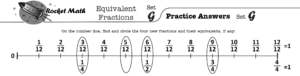EQUIVALENT FRACTIONS.  Students need to know that six-eighths is equivalent to three-fourths and that four-twelfths is equivalent to one-third.  While they can calculate these, it is very helpful to know the most common equivalent fractions by memory.  One of the most common problems students have in fractions is not “reducing their answers to simplest form.”  Equivalent fractions will help students commit 100 common equivalent fractions to memory.  Each set (A through Z) has four fractions which are displayed on a fraction number line.  Students frequently learn fractions equivalent to one,such as ten-tenths, as well as fractions that can’t be reduced, for example three-fourths is equivalent to three-fourths.  Using the fraction number line will help with student understanding of why those fractions are equivalent.

(Adding and subtracting positive and negative numbers).  Integers displays problems on a vertical number line and then teaches students two rules about how to solve problems that add or subtract positive and negative numbers.

Rule 1: Go up when you add a positive number OR subtract a negative number.
Rule 2: Go down when you subtract a positive number OR add a negative number.

Students gradually learn several variations of all four types of problems.  They practice with the number line on each page and then have a chance to build fluency on the top half of the page as they work with their partner.  You will probably not be surprised that there is a one-minute test on each set.  The goals are slightly different than before.  Students are to be 100% accurate and to complete at least 80% of their rate at answering simple addition and subtraction problems.

10s, 11s, 12s Multiplication and 10s, 11s, 12s Division facts are also available in the Universal Subscription.  If you have students who think they know the basic facts, but need review, putting them into either of these programs will review the 1s through 9s facts, teach them new ones and allow them to save face.

Among these five programs there are good things for ALL middle school math students to learn, even the more advanced students.  This will enable a math teacher to devote ten minutes a day to fact practice without holding anyone back.  Everyone will have something meaningful to practice during that time.  I think this could be a huge step forward for a lot of middle school MATH classrooms.

## What’s wrong with this picture?If you are seeing this in your school, you need Rocket Math!

Recently I gave my pre-service student teachers at Portland State University an assignment to do screening tests of basic skills in their placements. I was shocked to see how few of the screening tests showed students who were fluent with basic, single-digit math facts, where they could answer math facts as quickly as they could write. When children cannot answer math facts quickly and easily they are placed at a unnecessary disadvantage when it comes to doing math.

It is true that learning math facts takes time. No one can learn all of them in a matter of a few days or a week. It takes most students daily practice for months to learn all the facts in an operation. But when you consider that we require students to attend school five hours a day for years and years, it is pretty shocking to realize how many children do not have fluent mastery of math facts when they get to middle school. When the job can be done in ten minutes a day, and every child could become fluent in all four operations of addition, subtraction, multiplication and division by the end of fourth grade, why isn’t it?

Sometimes, teachers have been taught in their schools of education that helping children memorize things is somehow harmful. With that belief, teachers won’t try to do something systematic like Rocket Math. But after a year or two teaching, especially upper elementary grades, and struggling to teach higher math concepts to children who are interrupted by finger counting in the middle of every single computation, teachers learn that belief is simply wrong. Children are helped immensely by memorizing basic math facts. It enables them to have “number sense,” to easily appreciate the relationships among numerals, and to easily do computation.

Probably the main reason more students are not taught math facts, to the level they need, is that teachers are not aware of a tool that can help them do that. They don’t know that students enjoy doing learning math facts when it is done right. They don’t know that it can be done as a simple routine that takes ten minutes a day. They don’t know how easily students can master all of the facts. In short, they don’t know that Rocket Math exists. Someday a friend of theirs will tell them, because that is how Rocket Math spreads–by word-of-mouth.

If you read this, and you have never seen Rocket Math in action, you may be skeptical. Tell you what, write to me and if you need to see it in action to believe me, and don’t have a friend using Rocket Math, I’ll send you a free subscription to try it out.